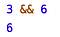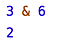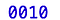So far the two programming languages we have worked with are Ruby and Javascript. There are many differences between the two, but one thing they have in common is how they deal with logical operators. AND is represented by &&, OR is represented with ||, etc. We are accustomed to using double symbol operators like && and ||. Why can’t we use use single symbols? Because the single symbol is reserved for bitwise operators.

What are bitwise operators? They are a way to interact with variables at the bit level. Bits are usually translated into floats and integers so it’s easier to digest the information. If we value speed and efficiency, then it will be useful to deal with bits directly and skip that translation into floats/ints. Bits are faster than variables in Javascript, but it seems like it’s more complicated than just skipping a level of translation.

Simple numbers in bits

1 (integer) = 0001 (binary)

2 = 0010

3 = 0011

4 = 0100

5 = 0101

6 = 0110

7 = 0111

8 = 1000

Bitwise operators (AND, OR, XOR) behave similarly to normal logic operators, except that they evaluate on a bit level and not the way we normally interpret logic. Here is an example of the difference between a bitwise operator and a normal logic operator.normal logic operators return the rightmost value when both are found to be truebitwise operators evaluate 3 AND 6 at a different level than normal logic operators

How can the result of 3 & 6 possibly equal 2? We see 3 & 6, but it really is

The result is 2 because the common denominator of those two bits is the second digit.The common digit is the second from the right, this bit represents the number 2

It’s clear that bitwise operators work on a different level than what we are accustomed to. Is there any benefit from using bitwise operators? It would be useful to find some practical applications for these operations. Evaluating at the bit level is faster than a normal logic operator, so evaluating or iterating over a large sample would be more efficient in bitwise operations. Another example that is more applicable to web development, is masking. We can do this! I think

Masking is a way to send a very simple string of bits, and allocate different digits to indicate different flags. This is a method to quickly ask a series of yes or no questions. Let’s pretend we have a website and we want to have 4 simple flags follow the user.

Flag A = “Is the user authenticated?” = 1

Flag B = “Is the user in the correct region?” = 2

Flag C = “Can we get ice cream after?” = 4

Flag D = “Is the user a robot?” = 8

These flags can be passed in a four digit binary string.

0000 = DCBA

By placing a 1 in the corresponding digit, we can raise the flags we want

1000 (binary) = Flag D = 8 (integer)

0100 (binary) = Flag C = 4 (integer)

0010 (binary)= Flag B = 2 (integer)

0001 (binary)= Flag A = 1 (integer)

This is a way to raise multiple flags at the same time when all we are passing through is an integer.

1010 (binary) = Flag D and Flag B = 10 (integer)

0111 (binary) = Flag C, B and A = 7 (integer)

Let’s make sure this works.

Cool, it worked. Totally expected that. Haha no I didn’t, but it’s great to see that we can pass an integer and raise multiple flags from it.

## bother7-blog

#### My coding blog

Welcome to a place where words matter. On Medium, smart voices and original ideas take center stage - with no ads in sight. Watch
Follow all the topics you care about, and we’ll deliver the best stories for you to your homepage and inbox. Explore
Get unlimited access to the best stories on Medium — and support writers while you’re at it. Just \$5/month. Upgrade